Filter
(found 1157 products)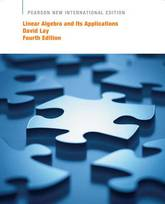Were you looking for the book with access to MyMathLab? This product is the book alone, and does NOT come with access to MyMathLab. Buy Linear Algebra and Its Applications with MyMathLab access card 4e (ISBN 9781447964209) if you need access to the MyLab as well, and save money on ...
Linear Algebra and Its Applications
111.56 USD

## Linear Algebra and Its Applications

by David C. Lay
Paperback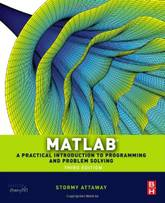MATLAB has become the standard software tool for solving scientific and engineering problems due to its powerful built-in functions and its ability to program. Assuming no knowledge of programming, this book guides the reader through both programming and built-in functions to easily exploit MATLAB's extensive capabilities for tackling engineering problems. ...
Matlab: A Practical Introduction to Programming and Problem Solving
MATLAB has become the standard software tool for solving scientific and engineering problems due to its powerful built-in functions and its ability to program. Assuming no knowledge of programming, this book guides the reader through both programming and built-in functions to easily exploit MATLAB's extensive capabilities for tackling engineering problems. The book starts with programming concepts, such as variables, assignments, and selection statements, moves on to loops, and then solves problems using both the programming concept and the power of MATLAB. In-depth coverage is given to input/output, a topic fundamental to many engineering applications. The third edition of MATLAB: A Practical Introduction to Programming and Problem Solving has been updated to reflect the functionality of the current version of MATLAB. It features new and revised end-of-chapter exercises, stronger coverage of loops and vectorizing, and more engineering applications to help the reader learn this software tool in context. It presents programming concepts and MATLAB built-in functions side-by-side. It is a systematic, step-by-step approach, building on concepts throughout the book, facilitating easier learning. It includes sections on common pitfalls and programming guidelines direct students towards best practice.
63.21 USD

## Matlab: A Practical Introduction to Programming and Problem Solving

by Stormy Attaway
Paperback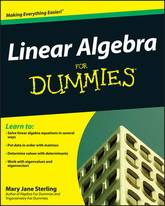An easy-to-follow guide to practical, real-world applications of linear algebra Linear algebra is a branch of mathematics that uses matrices to solve systems of linear equations; it has applications in many disciplines, from sociology and game theory to computer programming, engineering, and business. Linear Algebra For Dummies maps to a ...
Linear Algebra For Dummies
An easy-to-follow guide to practical, real-world applications of linear algebra Linear algebra is a branch of mathematics that uses matrices to solve systems of linear equations; it has applications in many disciplines, from sociology and game theory to computer programming, engineering, and business. Linear Algebra For Dummies maps to a typical, college-level linear algebra course, in which students first study matrices and matrix operations, then apply those fundamentals to abstract topics such as vector spaces, linear transformations, determinants, and, finally, eigenvalues and eigenvectors. It gives students theoretical and practical ways of approaching various types of problems, presenting the information in a way that allows readers to fully digest not just the how of solving linear algebraic problems, but also the why. Mary Jane Sterling (Peoria, IL) is the author of Algebra For Dummies (978-0-7645-5325-7), Business Math for Dummies (978-0-470-23331-3), and several other books. She has been teaching at Bradley University for almost 30 years.
20.990000 USD

## Linear Algebra For Dummies

by Mary Jane Sterling
Paperback / softback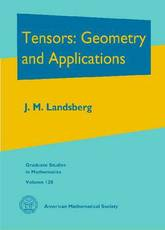Tensors are ubiquitous in the sciences. The geometry of tensors is both a powerful tool for extracting information from data sets, and a beautiful subject in its own right. This book has three intended uses: a classroom textbook, a reference work for researchers in the sciences, and an account of ...
Tensors: Geometry and Applications
Tensors are ubiquitous in the sciences. The geometry of tensors is both a powerful tool for extracting information from data sets, and a beautiful subject in its own right. This book has three intended uses: a classroom textbook, a reference work for researchers in the sciences, and an account of classical and modern results in (aspects of) the theory that will be of interest to researchers in geometry. For classroom use, there is a modern introduction to multilinear algebra and to the geometry and representation theory needed to study tensors, including a large number of exercises. For researchers in the sciences, there is information on tensors in table format for easy reference and a summary of the state of the art in elementary language. This is the first book containing many classical results regarding tensors. Particular applications treated in the book include the complexity of matrix multiplication, $\mathbf{P}$ versus $\mathbf{NP}$, signal processing, phylogenetics, and algebraic statistics. For geometers, there is material on secant varieties, $G$-varieties, spaces with finitely many orbits and how these objects arise in applications, discussions of numerous open questions in geometry arising in applications, and expositions of advanced topics such as the proof of the Alexander-Hirschowitz theorem and of the Weyman-Kempf method for computing syzygies.
140.41 USD

## Tensors: Geometry and Applications

by J. M. Landsberg
Hardback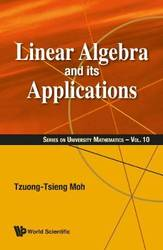From Tzuong-Tsieng Moh, a long-time expert in algebra, comes a new book for students to better understand linear algebra. Writing from an experienced standpoint, Moh touches on the many facets surrounding linear algebra, including but not limited to, echelon forms, matrix algebra, linear transformations, determinants, dual space, inner products, the ...
Linear Algebra And Its Applications
From Tzuong-Tsieng Moh, a long-time expert in algebra, comes a new book for students to better understand linear algebra. Writing from an experienced standpoint, Moh touches on the many facets surrounding linear algebra, including but not limited to, echelon forms, matrix algebra, linear transformations, determinants, dual space, inner products, the Gram-Schmidt Theorem, Hilbert space, and more. It is ideal for both newcomers and seasoned readers who want to attain a deeper understanding on both the basics and advanced topics of linear algebra and its vast applications. The wide range of topics combined with the depth of each discussion make it essential to be on the shelf of every mathematical beginner and enthusiast.
102.900000 USD

## Linear Algebra And Its Applications

by T.T. Moh
Hardback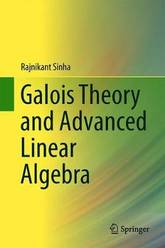This book discusses major topics in Galois theory and advanced linear algebra, including canonical forms. Divided into four chapters and presenting numerous new theorems, it serves as an easy-to-understand textbook for undergraduate students of advanced linear algebra, and helps students understand other courses, such as Riemannian geometry. The book also ...
Galois Theory and Advanced Linear Algebra
This book discusses major topics in Galois theory and advanced linear algebra, including canonical forms. Divided into four chapters and presenting numerous new theorems, it serves as an easy-to-understand textbook for undergraduate students of advanced linear algebra, and helps students understand other courses, such as Riemannian geometry. The book also discusses key topics including Cayley-Hamilton theorem, Galois groups, Sylvester's law of inertia, Eisenstein criterion, and solvability by radicals. Readers are assumed to have a grasp of elementary properties of groups, rings, fields, and vector spaces, and familiarity with the elementary properties of positive integers, inner product space of finite dimension and linear transformations is beneficial.
57.740000 USD

## Galois Theory and Advanced Linear Algebra

by Rajnikant Sinha
Hardback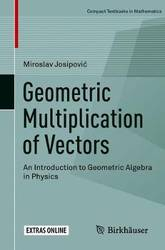This book enables the reader to discover elementary concepts of geometric algebra and its applications with lucid and direct explanations. Why would one want to explore geometric algebra? What if there existed a universal mathematical language that allowed one: to make rotations in any dimension with simple formulas, to see ...
Geometric Multiplication of Vectors: An Introduction to Geometric Algebra in Physics
This book enables the reader to discover elementary concepts of geometric algebra and its applications with lucid and direct explanations. Why would one want to explore geometric algebra? What if there existed a universal mathematical language that allowed one: to make rotations in any dimension with simple formulas, to see spinors or the Pauli matrices and their products, to solve problems of the special theory of relativity in three-dimensional Euclidean space, to formulate quantum mechanics without the imaginary unit, to easily solve difficult problems of electromagnetism, to treat the Kepler problem with the formulas for a harmonic oscillator, to eliminate unintuitive matrices and tensors, to unite many branches of mathematical physics? What if it were possible to use that same framework to generalize the complex numbers or fractals to any dimension, to play with geometry on a computer, as well as to make calculations in robotics, ray-tracing and brain science? In addition, what if such a language provided a clear, geometric interpretation of mathematical objects, even for the imaginary unit in quantum mechanics? Such a mathematical language exists and it is called geometric algebra. High school students have the potential to explore it, and undergraduate students can master it. The universality, the clear geometric interpretation, the power of generalizations to any dimension, the new insights into known theories, and the possibility of computer implementations make geometric algebra a thrilling field to unearth.
52.490000 USD

## Geometric Multiplication of Vectors: An Introduction to Geometric Algebra in Physics

by Miroslav Josipovic
Paperback / softback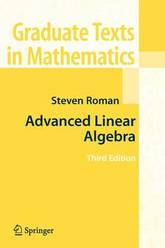This graduate level textbook covers an especially broad range of topics. The book first offers a careful discussion of the basics of linear algebra. It then proceeds to a discussion of modules, emphasizing a comparison with vector spaces, and presents a thorough discussion of inner product spaces, eigenvalues, eigenvectors, and ...
This graduate level textbook covers an especially broad range of topics. The book first offers a careful discussion of the basics of linear algebra. It then proceeds to a discussion of modules, emphasizing a comparison with vector spaces, and presents a thorough discussion of inner product spaces, eigenvalues, eigenvectors, and finite dimensional spectral theory, culminating in the finite dimensional spectral theorem for normal operators. The new edition has been revised and contains a chapter on the QR decomposition, singular values and pseudoinverses, and a chapter on convexity, separation and positive solutions to linear systems.
78.700000 USD

by Steven Roman
Paperback / softback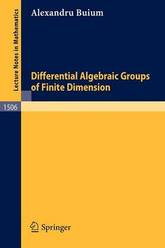Differential algebraic groups were introduced by P. Cassidy and E. Kolchin and are, roughly speaking, groups defined by algebraic differential equations in the same way as algebraic groups are groups defined by algebraic equations. The aim of the book is two-fold: 1) the provide an algebraic geometer's introduction to differential ...
Differential Algebraic Groups of Finite Dimension
Differential algebraic groups were introduced by P. Cassidy and E. Kolchin and are, roughly speaking, groups defined by algebraic differential equations in the same way as algebraic groups are groups defined by algebraic equations. The aim of the book is two-fold: 1) the provide an algebraic geometer's introduction to differential algebraic groups and 2) to provide a structure and classification theory for the finite dimensional ones. The main idea of the approach is to relate this topic to the study of: a) deformations of (not necessarily linear) algebraic groups and b) deformations of their automorphisms. The reader is assumed to possesssome standard knowledge of algebraic geometry but no familiarity with Kolchin's work is necessary. The book is both a research monograph and an introduction to a new topic and thus will be of interest to a wide audience ranging from researchers to graduate students.
41.950000 USD

## Differential Algebraic Groups of Finite Dimension

by Alexandru Buium
Paperback / softback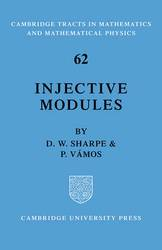In the preface of this book, the authors express the view that 'a good working knowledge of injective modules is a sound investment for module theorists'. The existing literature on the subject has tended to deal with the applications of injective modules to ring theory. The aim of this tract ...
Injective Modules
In the preface of this book, the authors express the view that 'a good working knowledge of injective modules is a sound investment for module theorists'. The existing literature on the subject has tended to deal with the applications of injective modules to ring theory. The aim of this tract is to demonstrate some of the applications of injective modules to commutative algebra. A number of well-known concepts and results which so far have been applicable principally to commutative rings are generalized to a non-commutative context. There are exercises and brief notes appended to each chapter to illustrate and extend the scope of the treatment in the main text. Together with the short bibliography the notes form a guide to sources of reading for students and researchers who wish to delve more exhaustively into the theory of injective modules. The tract is intended primarily for those who have some knowledge of the rudiments of commutative algebra, although these are recalled at the outset.
74.37 USD

## Injective Modules

by Sharpe
Paperback / softback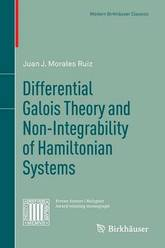This book is devoted to the relation between two different concepts of integrability: the complete integrability of complex analytical Hamiltonian systems and the integrability of complex analytical linear differential equations. For linear differential equations, integrability is made precise within the framework of differential Galois theory. The connection of these two ...
Differential Galois Theory and Non-Integrability of Hamiltonian Systems
This book is devoted to the relation between two different concepts of integrability: the complete integrability of complex analytical Hamiltonian systems and the integrability of complex analytical linear differential equations. For linear differential equations, integrability is made precise within the framework of differential Galois theory. The connection of these two integrability notions is given by the variational equation (i.e. linearized equation) along a particular integral curve of the Hamiltonian system. The underlying heuristic idea, which motivated the main results presented in this monograph, is that a necessary condition for the integrability of a Hamiltonian system is the integrability of the variational equation along any of its particular integral curves. This idea led to the algebraic non-integrability criteria for Hamiltonian systems. These criteria can be considered as generalizations of classical non-integrability results by Poincare and Lyapunov, as well as more recent results by Ziglin and Yoshida. Thus, by means of the differential Galois theory it is not only possible to understand all these approaches in a unified way but also to improve them. Several important applications are also included: homogeneous potentials, Bianchi IX cosmological model, three-body problem, Henon-Heiles system, etc. The book is based on the original joint research of the author with J.M. Peris, J.P. Ramis and C. Simo, but an effort was made to present these achievements in their logical order rather than their historical one. The necessary background on differential Galois theory and Hamiltonian systems is included, and several new problems and conjectures which open new lines of research are proposed. - - - The book is an excellent introduction to non-integrability methods in Hamiltonian mechanics and brings the reader to the forefront of research in the area. The inclusion of a large number of worked-out examples, many of wide applied interest, is commendable. There are many historical references, and an extensive bibliography. (Mathematical Reviews) For readers already prepared in the two prerequisite subjects [differential Galois theory and Hamiltonian dynamical systems], the author has provided a logically accessible account of a remarkable interaction between differential algebra and dynamics. (Zentralblatt MATH)
83.990000 USD

## Differential Galois Theory and Non-Integrability of Hamiltonian Systems

by Juan J. Morales Ruiz
Paperback / softback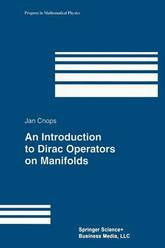The chapters on Clifford algebra and differential geometry can be used as an introduction to the topics, and are suitable for senior undergraduates and graduates. The other chapters are also accessible at this level.; This self-contained book requires very little previous knowledge of the domains covered, although the reader will ...
An Introduction to Dirac Operators on Manifolds
The chapters on Clifford algebra and differential geometry can be used as an introduction to the topics, and are suitable for senior undergraduates and graduates. The other chapters are also accessible at this level.; This self-contained book requires very little previous knowledge of the domains covered, although the reader will benefit from knowledge of complex analysis, which gives the basic example of a Dirac operator.; The more advanced reader will appreciate the fresh approach to the theory, as well as the new results on boundary value theory.; Concise, but self-contained text at the introductory grad level. Systematic exposition.; Clusters well with other Birkhauser titles in mathematical physics.; Appendix. General Manifolds * List of Symbols * Bibliography * Index
103.950000 USD

## An Introduction to Dirac Operators on Manifolds

by Jan Cnops
Paperback / softback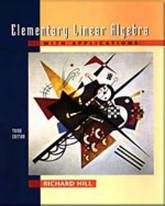This edition strives to develop students' geometric intuition as a foundation for learning the concepts of span and linear independence. Applications are integrated throughout to illustrate the mathematics and to motivate the student. Numerical ideas and concepts using the computer are interspersed throughout the text; instructors can use these at ...
Elementary Linear Algebra with Applications
This edition strives to develop students' geometric intuition as a foundation for learning the concepts of span and linear independence. Applications are integrated throughout to illustrate the mathematics and to motivate the student. Numerical ideas and concepts using the computer are interspersed throughout the text; instructors can use these at their discretion. This textbook allows the instructor considerable flexibility to choose the applications and numerical topics to be covered according to his or her tastes and the students' needs.
348.550000 USD

## Elementary Linear Algebra with Applications

by Richard O. Hill
Hardback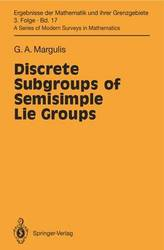A detailed treatment of the geometric aspects of discrete groups was carried out by Raghunathan in his book Discrete subgroups of Lie Groups which appeared in 1972. In particular he covered the theory of lattices in nilpotent and solvable Lie groups, results of Mal'cev and Mostow, and proved the Borel ...
Discrete Subgroups of Semisimple Lie Groups
A detailed treatment of the geometric aspects of discrete groups was carried out by Raghunathan in his book Discrete subgroups of Lie Groups which appeared in 1972. In particular he covered the theory of lattices in nilpotent and solvable Lie groups, results of Mal'cev and Mostow, and proved the Borel density theorem and local rigidity theorem ofSelberg-Weil. He also included some results on unipotent elements of discrete subgroups as well as on the structure of fundamental domains. The chapters concerning discrete subgroups of semi simple Lie groups are essentially concerned with results which were obtained in the 1960's. The present book is devoted to lattices, i.e. discrete subgroups of finite covolume, in semi-simple Lie groups. By Lie groups we not only mean real Lie groups, but also the sets of k-rational points of algebraic groups over local fields k and their direct products. Our results can be applied to the theory of algebraic groups over global fields. For example, we prove what is in some sense the best possible classification of abstract homomorphisms of semi-simple algebraic group over global fields.
230.990000 USD

## Discrete Subgroups of Semisimple Lie Groups

by Gregori A. Margulis
Hardback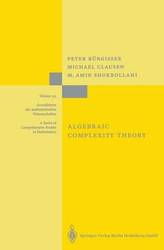The algorithmic solution of problems has always been one of the major concerns of mathematics. For a long time such solutions were based on an intuitive notion of algorithm. It is only in this century that metamathematical problems have led to the intensive search for a precise and sufficiently general ...
Algebraic Complexity Theory
The algorithmic solution of problems has always been one of the major concerns of mathematics. For a long time such solutions were based on an intuitive notion of algorithm. It is only in this century that metamathematical problems have led to the intensive search for a precise and sufficiently general formalization of the notions of computability and algorithm. In the 1930s, a number of quite different concepts for this purpose were pro posed, such as Turing machines, WHILE-programs, recursive functions, Markov algorithms, and Thue systems. All these concepts turned out to be equivalent, a fact summarized in Church's thesis, which says that the resulting definitions form an adequate formalization of the intuitive notion of computability. This had and continues to have an enormous effect. First of all, with these notions it has been possible to prove that various problems are algorithmically unsolvable. Among of group these undecidable problems are the halting problem, the word problem theory, the Post correspondence problem, and Hilbert's tenth problem. Secondly, concepts like Turing machines and WHILE-programs had a strong influence on the development of the first computers and programming languages. In the era of digital computers, the question of finding efficient solutions to algorithmically solvable problems has become increasingly important. In addition, the fact that some problems can be solved very efficiently, while others seem to defy all attempts to find an efficient solution, has called for a deeper under standing of the intrinsic computational difficulty of problems.
125.990000 USD

## Algebraic Complexity Theory

by Mohammad A. Shokrollahi, Michael Clausen, Peter Burgisser
Hardback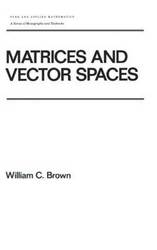A textbook for a one-semester course in linear algebra for graduate or upper-level undergraduate students of mathematics and engineering. Employs a matrix perspective, and emphasizes training in definitions, theorems, and proofs. Annotation copyright Book News, Inc. Portland, Or.
Matrices and Vector SPates
A textbook for a one-semester course in linear algebra for graduate or upper-level undergraduate students of mathematics and engineering. Employs a matrix perspective, and emphasizes training in definitions, theorems, and proofs. Annotation copyright Book News, Inc. Portland, Or.
126.000000 USD

## Matrices and Vector SPates

by William C Brown
Hardback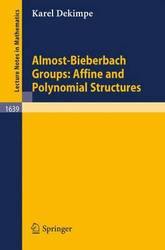Starting from basic knowledge of nilpotent (Lie) groups, an algebraic theory of almost-Bieberbach groups, the fundamental groups of infra-nilmanifolds, is developed. These are a natural generalization of the well known Bieberbach groups and many results about ordinary Bieberbach groups turn out to generalize to the almost-Bieberbach groups. Moreover, using affine ...
Almost-Bieberbach Groups: Affine and Polynomial Structures
Starting from basic knowledge of nilpotent (Lie) groups, an algebraic theory of almost-Bieberbach groups, the fundamental groups of infra-nilmanifolds, is developed. These are a natural generalization of the well known Bieberbach groups and many results about ordinary Bieberbach groups turn out to generalize to the almost-Bieberbach groups. Moreover, using affine representations, explicit cohomology computations can be carried out, or resulting in a classification of the almost-Bieberbach groups in low dimensions. The concept of a polynomial structure, an alternative for the affine structures that sometimes fail, is introduced.
73.450000 USD

## Almost-Bieberbach Groups: Affine and Polynomial Structures

by Karel Dekimpe
Paperback / softback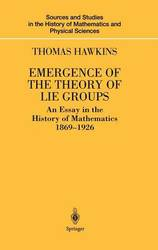The great Norwegian mathematician Sophus Lie developed the general theory of transformations in the 1870s, and the first part of the book properly focuses on his work. In the second part the central figure is Wilhelm Killing, who developed structure and classification of semisimple Lie algebras. The third part focuses ...
Emergence of the Theory of Lie Groups: An Essay in the History of Mathematics 1869-1926
The great Norwegian mathematician Sophus Lie developed the general theory of transformations in the 1870s, and the first part of the book properly focuses on his work. In the second part the central figure is Wilhelm Killing, who developed structure and classification of semisimple Lie algebras. The third part focuses on the developments of the representation of Lie algebras, in particular the work of Elie Cartan. The book concludes with the work of Hermann Weyl and his contemporaries on the structure and representation of Lie groups which serves to bring together much of the earlier work into a coherent theory while at the same time opening up significant avenues for further work.
209.990000 USD

## Emergence of the Theory of Lie Groups: An Essay in the History of Mathematics 1869-1926

by Thomas Hawkins
Hardback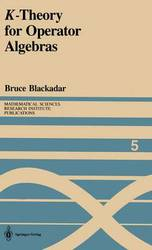K -Theory has revolutionized the study of operator algebras in the last few years. As the primary component of the subject of noncommutative topol- ogy, K -theory has opened vast new vistas within the structure theory of C*- algebras, as well as leading to profound and unexpected applications of opera- ...
K-Theory for Operator Algebras
K -Theory has revolutionized the study of operator algebras in the last few years. As the primary component of the subject of noncommutative topol- ogy, K -theory has opened vast new vistas within the structure theory of C*- algebras, as well as leading to profound and unexpected applications of opera- tor algebras to problems in geometry and topology. As a result, many topolo- gists and operator algebraists have feverishly begun trying to learn each others' subjects, and it appears certain that these two branches of mathematics have become deeply and permanently intertwined. Despite the fact that the whole subject is only about a decade old, operator K -theory has now reached a state of relative stability. While there will undoubtedly be many more revolutionary developments and applications in the future, it appears the basic theory has more or less reached a final form. But because of the newness of the theory, there has so far been no comprehensive treatment of the subject. It is the ambitious goal of these notes to fill this gap. We will develop the K -theory of Banach algebras, the theory of extensions of C*-algebras, and the operator K -theory of Kasparov from scratch to its most advanced aspects. We will not treat applications in detail; however, we will outline the most striking of the applications to date in a section at the end, as well as mentioning others at suitable points in the text.
146.990000 USD

## K-Theory for Operator Algebras

Paperback / softback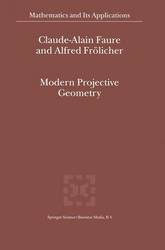This monograph develops projective geometries and provides a systematic treatment of morphisms. It introduces a new fundamental theorem and its applications describing morphisms of projective geometries in homogeneous coordinates by semilinear maps. Other topics treated include three equivalent definitions of projective geometries and their correspondence with certain lattices; quotients of ...
Modern Projective Geometry
This monograph develops projective geometries and provides a systematic treatment of morphisms. It introduces a new fundamental theorem and its applications describing morphisms of projective geometries in homogeneous coordinates by semilinear maps. Other topics treated include three equivalent definitions of projective geometries and their correspondence with certain lattices; quotients of projective geometries and isomorphism theorems; and recent results in dimension theory.
230.990000 USD

## Modern Projective Geometry

by Alfred Frolicher, Claude-Alain Faure
Paperback / softback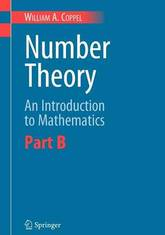This two-volume book is a modern introduction to the theory of numbers, emphasizing its connections with other branches of mathematics. Part A is accessible to first-year undergraduates and deals with elementary number theory. Part B is more advanced and gives the reader an idea of the scope of mathematics today. ...
Number Theory: An Introduction to Mathematics: Part B
This two-volume book is a modern introduction to the theory of numbers, emphasizing its connections with other branches of mathematics. Part A is accessible to first-year undergraduates and deals with elementary number theory. Part B is more advanced and gives the reader an idea of the scope of mathematics today. The connecting theme is the theory of numbers. By exploring its many connections with other branches a broad picture is obtained. The book contains a treasury of proofs, several of which are gems seldom seen in number theory books.
52.450000 USD

## Number Theory: An Introduction to Mathematics: Part B

by W.A. Coppel
Paperback / softback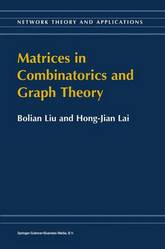Combinatorics and Matrix Theory have a symbiotic, or mutually beneficial, relationship. This relationship is discussed in my paper The symbiotic relationship of combinatorics and matrix theoryl where I attempted to justify this description. One could say that a more detailed justification was given in my book with H. J. Ryser ...
Matrices in Combinatorics and Graph Theory
Combinatorics and Matrix Theory have a symbiotic, or mutually beneficial, relationship. This relationship is discussed in my paper The symbiotic relationship of combinatorics and matrix theoryl where I attempted to justify this description. One could say that a more detailed justification was given in my book with H. J. Ryser entitled Combinatorial Matrix Theon? where an attempt was made to give a broad picture of the use of combinatorial ideas in matrix theory and the use of matrix theory in proving theorems which, at least on the surface, are combinatorial in nature. In the book by Liu and Lai, this picture is enlarged and expanded to include recent developments and contributions of Chinese mathematicians, many of which have not been readily available to those of us who are unfamiliar with Chinese journals. Necessarily, there is some overlap with the book Combinatorial Matrix Theory. Some of the additional topics include: spectra of graphs, eulerian graph problems, Shannon capacity, generalized inverses of Boolean matrices, matrix rearrangements, and matrix completions. A topic to which many Chinese mathematicians have made substantial contributions is the combinatorial analysis of powers of nonnegative matrices, and a large chapter is devoted to this topic. This book should be a valuable resource for mathematicians working in the area of combinatorial matrix theory. Richard A. Brualdi University of Wisconsin - Madison 1 Linear Alg. Applies., vols. 162-4, 1992, 65-105 2Camhridge University Press, 1991.
178.490000 USD

## Matrices in Combinatorics and Graph Theory

by Hong-Jian Lai, Bolian Liu
Paperback / softback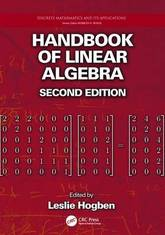With a substantial amount of new material, the Handbook of Linear Algebra, Second Edition provides comprehensive coverage of linear algebra concepts, applications, and computational software packages in an easy-to-use format. It guides you from the very elementary aspects of the subject to the frontiers of current research. Along with revisions ...
Handbook of Linear Algebra
With a substantial amount of new material, the Handbook of Linear Algebra, Second Edition provides comprehensive coverage of linear algebra concepts, applications, and computational software packages in an easy-to-use format. It guides you from the very elementary aspects of the subject to the frontiers of current research. Along with revisions and updates throughout, the second edition of this bestseller includes 20 new chapters. New to the Second Edition Separate chapters on Schur complements, additional types of canonical forms, tensors, matrix polynomials, matrix equations, special types of matrices, generalized inverses, matrices over finite fields, invariant subspaces, representations of quivers, and spectral sets New chapters on combinatorial matrix theory topics, such as tournaments, the minimum rank problem, and spectral graph theory, as well as numerical linear algebra topics, including algorithms for structured matrix computations, stability of structured matrix computations, and nonlinear eigenvalue problems More chapters on applications of linear algebra, including epidemiology and quantum error correction New chapter on using the free and open source software system Sage for linear algebra Additional sections in the chapters on sign pattern matrices and applications to geometry Conjectures and open problems in most chapters on advanced topics Highly praised as a valuable resource for anyone who uses linear algebra, the first edition covered virtually all aspects of linear algebra and its applications. This edition continues to encompass the fundamentals of linear algebra, combinatorial and numerical linear algebra, and applications of linear algebra to various disciplines while also covering up-to-date software packages for linear algebra computations.
105.99 USD

## Handbook of Linear Algebra

Paperback / softback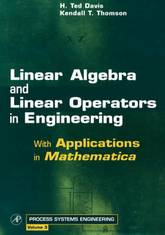Designed for advanced engineering, physical science, and applied mathematics students, this innovative textbook is an introduction to both the theory and practical application of linear algebra and functional analysis. The book is self-contained, beginning with elementary principles, basic concepts, and definitions. The important theorems of the subject are covered and ...
Linear Algebra and Linear Operators in Engineering: With Applications in Mathematica (R): Volume 3
Designed for advanced engineering, physical science, and applied mathematics students, this innovative textbook is an introduction to both the theory and practical application of linear algebra and functional analysis. The book is self-contained, beginning with elementary principles, basic concepts, and definitions. The important theorems of the subject are covered and effective application tools are developed, working up to a thorough treatment of eigenanalysis and the spectral resolution theorem. Building on a fundamental understanding of finite vector spaces, infinite dimensional Hilbert spaces are introduced from analogy. Wherever possible, theorems and definitions from matrix theory are called upon to drive the analogy home. The result is a clear and intuitive segue to functional analysis, culminating in a practical introduction to the functional theory of integral and differential operators. Numerous examples, problems, and illustrations highlight applications from all over engineering and the physical sciences. Also included are several numerical applications, complete with Mathematica solutions and code, giving the student a hands-on introduction to numerical analysis. Linear Algebra and Linear Operators in Engineering is ideally suited as the main text of an introductory graduate course, and is a fine instrument for self-study or as a general reference for those applying mathematics.
168.000000 USD

## Linear Algebra and Linear Operators in Engineering: With Applications in Mathematica (R): Volume 3

by Kendall T. Thomson, H. Ted Davis
Hardback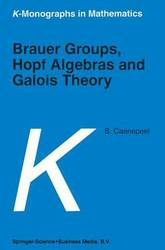This volume is devoted to the Brauer group of a commutative ring and related invariants. Part I presents a new self-contained exposition of the Brauer group of a commutative ring. Included is a systematic development of the theory of Grothendieck topologies and etale cohomology, and discussion of topics such as ...
Brauer Groups, Hopf Algebras and Galois Theory
This volume is devoted to the Brauer group of a commutative ring and related invariants. Part I presents a new self-contained exposition of the Brauer group of a commutative ring. Included is a systematic development of the theory of Grothendieck topologies and etale cohomology, and discussion of topics such as Gabber's theorem and the theory of Taylor's big Brauer group of algebras without a unit. Part II presents a systematic development of the Galois theory of Hopf algebras with special emphasis on the group of Galois objects of a cocommutative Hopf algebra. The development of the theory is carried out in such a way that the connection to the theory of the Brauer group in Part I is made clear. Recent developments are considered and examples are included. The Brauer-Long group of a Hopf algebra over a commutative ring is discussed in Part III. This provides a link between the first two parts of the volume and is the first time this topic has been discussed in a monograph. Audience: Researchers whose work involves group theory. The first two parts, in particular, can be recommended for supplementary, graduate course use.
94.490000 USD

## Brauer Groups, Hopf Algebras and Galois Theory

by Stefaan Caenepeel
Paperback / softback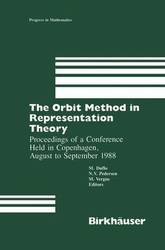Ever since its introduction around 1960 by Kirillov, the orbit method has played a major role in representation theory of Lie groups and Lie algebras. This book contains the proceedings of a conference held from August 29 to September 2, 1988, at the University of Copenhagen, about the orbit method ...
The Orbit Method in Representation Theory: Proceedings of a Conference Held in Copenhagen, August to September 1988
Ever since its introduction around 1960 by Kirillov, the orbit method has played a major role in representation theory of Lie groups and Lie algebras. This book contains the proceedings of a conference held from August 29 to September 2, 1988, at the University of Copenhagen, about the orbit method in representation theory. It contains ten articles, most of which are original research papers, by well-known mathematicians in the field, and it reflects the fact that the orbit method plays an important role in the representation theory of semisimple Lie groups, solvable Lie groups, and even more general Lie groups, and also in the theory of enveloping algebras.
125.990000 USD

## The Orbit Method in Representation Theory: Proceedings of a Conference Held in Copenhagen, August to September 1988

by Michele Vergne, N.V. Pedersen, Michel Duflo
Hardback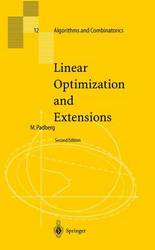From the reviews: Do you know M.Padberg's Linear Optimization and Extensions? [...] Now here is the continuation of it, discussing the solutions of all its exercises and with detailed analysis of the applications mentioned. Tell your students about it. [...] For those who strive for good exercises and case studies ...
Linear Optimization and Extensions
From the reviews: Do you know M.Padberg's Linear Optimization and Extensions? [...] Now here is the continuation of it, discussing the solutions of all its exercises and with detailed analysis of the applications mentioned. Tell your students about it. [...] For those who strive for good exercises and case studies for LP this is an excellent volume. Acta Scientiarum Mathematicarum
146.990000 USD

## Linear Optimization and Extensions

Hardback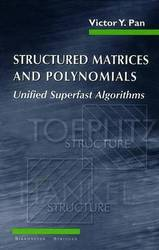This user-friendly, engaging textbook makes the material accessible to graduate students and new researchers who wish to study the rapidly exploding area of computations with structured matrices and polynomials. The book goes beyond research frontiers and, apart from very recent research articles, includes previously unpublished results.
Structured Matrices and Polynomials: Unified Superfast Algorithms
This user-friendly, engaging textbook makes the material accessible to graduate students and new researchers who wish to study the rapidly exploding area of computations with structured matrices and polynomials. The book goes beyond research frontiers and, apart from very recent research articles, includes previously unpublished results.
89.240000 USD

## Structured Matrices and Polynomials: Unified Superfast Algorithms

by Victor Y. Pan
Paperback / softback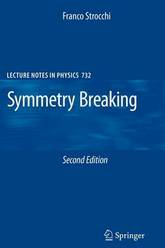The new edition of this well received primer on rigorous aspects of symmetry breaking presents a more detailed and thorough discussion of the mechanism of symmetry breaking in classical field theory in relation with the Noether theorem. Moreover, the link between symmetry breaking without massless Goldstone bosons in Coulomb systems ...
Symmetry Breaking
The new edition of this well received primer on rigorous aspects of symmetry breaking presents a more detailed and thorough discussion of the mechanism of symmetry breaking in classical field theory in relation with the Noether theorem. Moreover, the link between symmetry breaking without massless Goldstone bosons in Coulomb systems and in gauge theories is made more explicit. A subject index has been added and a number of misprints have been corrected.
78.740000 USD

## Symmetry Breaking

by Franco Strocchi
Paperback / softback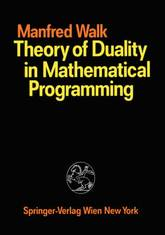Courses on mathematical programming are now part of standard teaching programs of universities and institutes. The aim of this book is to introduce students of mathematics, economics, technology and other related subjects to the qualitative theory of mathematical programming in paired vector spaces. Prerequisite for the study of this book ...
Theory of Duality in Mathematical Programming
Courses on mathematical programming are now part of standard teaching programs of universities and institutes. The aim of this book is to introduce students of mathematics, economics, technology and other related subjects to the qualitative theory of mathematical programming in paired vector spaces. Prerequisite for the study of this book is only a basic knowledge of analysis, of elements of functional analysis and linear algebra. The application of elementary ideas of functional analysis is convenient for a more rigorous construction of proofs and for some generalizations of the finite dimensional theory on infinite dimensional Banach-spaces. An important feature of this book is the use of a principle of duality to formulate the theoretical basis of many different concrete programming problems. The main idea of the book is to present relations of duality and to construct a general theoretical basis for different special programming problems.